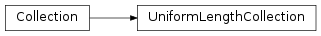# mvpa2.base.collections.UniformLengthCollection¶class `mvpa2.base.collections.``UniformLengthCollection`(items=None, length=None)

Container for attributes with the same length.

Attributes

 `attr_length` Uniform length of all attributes in a collection

Methods

`clear`(() -> None.  Remove all items from D.)
`copy`(\*args, \*\*kwargs) Create a copy of a collection.
`fromkeys`(...) v defaults to None.
`get`((k[,d]) -> D[k] if k in D, ...)
`has_key`((k) -> True if D has a key k, else False)
`items`(() -> list of D’s (key, value) pairs, ...)
`iteritems`(() -> an iterator over the (key, ...)
`iterkeys`(() -> an iterator over the keys of D)
`itervalues`(...)
`keys`(() -> list of D’s keys)
`match`(d[, strict]) Given a dictionary describing selection, return mask for matching items
`pop`((k[,d]) -> v, ...) If key is not found, d is returned if given, otherwise KeyError is raised
`popitem`(() -> (k, v), ...) 2-tuple; but raise KeyError if D is empty.
`set_length_check`(value)
`setdefault`((k[,d]) -> D.get(k,d), ...)
`update`(source[, copyvalues, memo])
`values`(() -> list of D’s values)
`viewitems`(...)
`viewkeys`(...)
`viewvalues`(...)
Parameters: length : int When adding items to the collection, they are checked if the have this length.

Attributes

 `attr_length` Uniform length of all attributes in a collection

Methods

`clear`(() -> None.  Remove all items from D.)
`copy`(\*args, \*\*kwargs) Create a copy of a collection.
`fromkeys`(...) v defaults to None.
`get`((k[,d]) -> D[k] if k in D, ...)
`has_key`((k) -> True if D has a key k, else False)
`items`(() -> list of D’s (key, value) pairs, ...)
`iteritems`(() -> an iterator over the (key, ...)
`iterkeys`(() -> an iterator over the keys of D)
`itervalues`(...)
`keys`(() -> list of D’s keys)
`match`(d[, strict]) Given a dictionary describing selection, return mask for matching items
`pop`((k[,d]) -> v, ...) If key is not found, d is returned if given, otherwise KeyError is raised
`popitem`(() -> (k, v), ...) 2-tuple; but raise KeyError if D is empty.
`set_length_check`(value)
`setdefault`((k[,d]) -> D.get(k,d), ...)
`update`(source[, copyvalues, memo])
`values`(() -> list of D’s values)
`viewitems`(...)
`viewkeys`(...)
`viewvalues`(...)
`attr_length`

Uniform length of all attributes in a collection

`copy`(*args, **kwargs)

Create a copy of a collection.

By default this is going to return a deep copy of the collection, hence no data would be shared between the original dataset and its copy.

Parameters: deep : boolean, optional If False, a shallow copy of the collection is return instead. The copy contains only views of the values. a : list or None List of attributes to include in the copy of the dataset. If `None` all attributes are considered. If an empty list is given, all attributes are stripped from the copy. memo : dict Developers only: This argument is only useful if copy() is called inside the __deepcopy__() method and refers to the dict-argument `memo` in the Python documentation.
`match`(d, strict=True)

Given a dictionary describing selection, return mask for matching items

Given a dictionary with keys known to the collection, search for item attributes which would satisfy the selection. E.g.

```>>> col = UniformLengthCollection({'roi': ['a', 'b', 'c', 'a']})
>>> print col.match({'roi': ['a']})
[ True False False  True]
>>> print col.match({'roi': ['c', 'a']})
[ True False  True  True]
```

Multiple keys could be specified with desired matching values. Intersection of matchings is returned across different keys:

```>>> col = UniformLengthCollection({'roi': ['a', 'b', 'c', 'a'],
...                                'vox': [[1,0], [0,1], [1,0], [0, 1]]})
>>> print col.match({'roi': ['c', 'a'], 'vox': [[0,1]]})
[False False False  True]
```
Parameters: d: dict Dict describing the selection. Keys must be known to the collection strict: bool, optional If True, absent matching to any specified selection key/value pair would result in ValueError exception. If False, it would allow to not have matches, but if only a single value for a key is given or none of the values match – you will end up with empty selection.
`set_length_check`(value)
Parameters: value : int When adding new items to the collection, they are checked if the have this length.